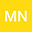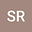A new regularization method for a parameter identification problem in a non-linear partial differential equation
••• M Thamban Nair,
• Samprita Roy
M Thamban NairWe consider a parameter identification problem associated with a quasi-linear elliptic Neumann boundary value problem involving a parameter function $$a(\cdot)$$ and the solution $$u(\cdot)$$, where the problem is to identify $$a(\cdot)$$ on an interval $$I:=g(\Gamma)$$ from the knowledge of the solution $$u(\cdot)$$ as $$g$$ on $$\Gamma$$, where $$\Gamma$$ is a given curve on the boundary of the domain $$\Omega\subseteq{\mathbb{R}}^{3}$$ of the problem and $$g$$ is a continuous function. The inverse problem is formulated as a problem of solving an operator equation involving a compact operator depending on the data, and for obtaining stable approximate solutions under noisy data, a new regularization method is considered. The derived error estimates are similar to, and in certain cases better than, the classical Tikhonov regularization considered in the literature in the recent past.